# Analyzing GRB 080916C¶

To demonstrate the capabilities and features of 3ML in, we will go through a time-integrated and time-resolved analysis. This example serves as a standard way to analyze Fermi-GBM data with 3ML as well as a template for how you can design your instrument’s analysis pipeline with 3ML if you have similar data.

:

# Scientific libraries
import numpy as np

import matplotlib.pyplot as plt
from jupyterthemes import jtplot

%matplotlib inline
jtplot.style(context="talk", fscale=1, ticks=True, grid=False)
plt.style.use("mike")

from threeML import *

import warnings

warnings.simplefilter("ignore")


## Examining the catalog¶

As with Swift and Fermi-LAT, 3ML provides a simple interface to the on-line Fermi-GBM catalog. Let’s get the information for GRB 080916C.

:

gbm_catalog = FermiGBMBurstCatalog()
gbm_catalog.query_sources("GRB080916009")

:

Table length=1
nameradectrigger_timet90
objectfloat64float64float64float64
GRB080916009119.800-56.60054725.008861362.977

To aid in quickly replicating the catalog analysis, and thanks to the tireless efforts of the Fermi-GBM team, we have added the ability to extract the analysis parameters from the catalog as well as build an astromodels model with the best fit parameters baked in. Using this information, one can quickly run through the catalog an replicate the entire analysis with a script. Let’s give it a try.

:

grb_info =  gbm_catalog.get_detector_information()["GRB080916009"]

gbm_detectors = grb_info['detectors']
source_interval = grb_info["source"]["fluence"]
background_interval = grb_info["background"]["full"]
best_fit_model = grb_info["best fit model"]["fluence"]
model =  gbm_catalog.get_model(best_fit_model, "fluence")["GRB080916009"]

:

model

:

Model summary:

N
Point sources 1
Extended sources 0
Particle sources 0

Free parameters (5):

value min_value max_value unit
GRB080916009.spectrum.main.SmoothlyBrokenPowerLaw.K 0.0122548 0 None keV-1 s-1 cm-2
GRB080916009.spectrum.main.SmoothlyBrokenPowerLaw.alpha -1.13042 -1.5 2
GRB080916009.spectrum.main.SmoothlyBrokenPowerLaw.break_energy 309.203 10 None keV
GRB080916009.spectrum.main.SmoothlyBrokenPowerLaw.break_scale 0.3 0 10
GRB080916009.spectrum.main.SmoothlyBrokenPowerLaw.beta -2.09693 -5 -1.6

Fixed parameters (3):
(abridged. Use complete=True to see all fixed parameters)

Linked parameters (0):

(none)

Independent variables:

(none)

## Downloading the data¶

We provide a simple interface to download the Fermi-GBM data. Using the information from the catalog that we have extracted, we can download just the data from the detectors that were used for the catalog analysis. This will download the CSPEC, TTE and instrument response files from the on-line database.

:

dload = download_GBM_trigger_data("bn080916009",detectors=gbm_detectors)


Let’s first examine the catalof fluence fit. Using the TimeSeriesBuilder, we can fit the background, set the source interval, and create a 3ML plugin for the analysis. We will loop through the detectors, set their appropriate channel selections, and ensure there are enough counts in each bin to make the PGStat profile likelihood valid.

• First we use the CSPEC data to fit the background using the background selections. We use CSPEC because it has a longer duration for fitting the background.

• The background is saved to an HDF5 file that stores the polynomial coefficients and selections which we can read in to the TTE file later.

• The light curve is plotted.

• The source selection from the catalog is set and DispersionSpectrumLike plugin is created.

• The plugin has the standard GBM channel selections for spectral analysis set.

:

fluence_plugins = []
time_series = {}
for det in gbm_detectors:

ts_cspec = TimeSeriesBuilder.from_gbm_cspec_or_ctime(
det, cspec_or_ctime_file=dload[det]["cspec"], rsp_file=dload[det]["rsp"]
)

ts_cspec.set_background_interval(*background_interval.split(","))
ts_cspec.save_background(f"{det}_bkg.h5", overwrite=True)

ts_tte = TimeSeriesBuilder.from_gbm_tte(
det,
tte_file=dload[det]["tte"],
rsp_file=dload[det]["rsp"],
restore_background=f"{det}_bkg.h5",
)

time_series[det] = ts_tte

ts_tte.set_active_time_interval(source_interval)

ts_tte.view_lightcurve(-40, 100)

fluence_plugin = ts_tte.to_spectrumlike()

if det.startswith("b"):

fluence_plugin.set_active_measurements("250-30000")

else:

fluence_plugin.set_active_measurements("9-900")

fluence_plugin.rebin_on_background(1.)

fluence_plugins.append(fluence_plugin)

Auto-determined polynomial order: 0


Binned 0-order polynomial fit with the Powell method

Saved fitted background to n3_bkg.h5.

Successfully restored fit from n3_bkg.h5
Auto-probed noise models:
- observation: poisson
- background: gaussian
Range 9-900 translates to channels 5-124
Now using 120 channels out of 128
Now using 120 bins

Auto-determined polynomial order: 1


Binned 1-order polynomial fit with the Powell method

Saved fitted background to n4_bkg.h5.

Successfully restored fit from n4_bkg.h5
Auto-probed noise models:
- observation: poisson
- background: gaussian
Range 9-900 translates to channels 5-123
Now using 119 channels out of 128
Now using 119 bins

Auto-determined polynomial order: 1


Binned 1-order polynomial fit with the Powell method

Saved fitted background to b0_bkg.h5.

Successfully restored fit from b0_bkg.h5
Auto-probed noise models:
- observation: poisson
- background: gaussian
Range 250-30000 translates to channels 1-119
Now using 119 channels out of 128
Now using 119 bins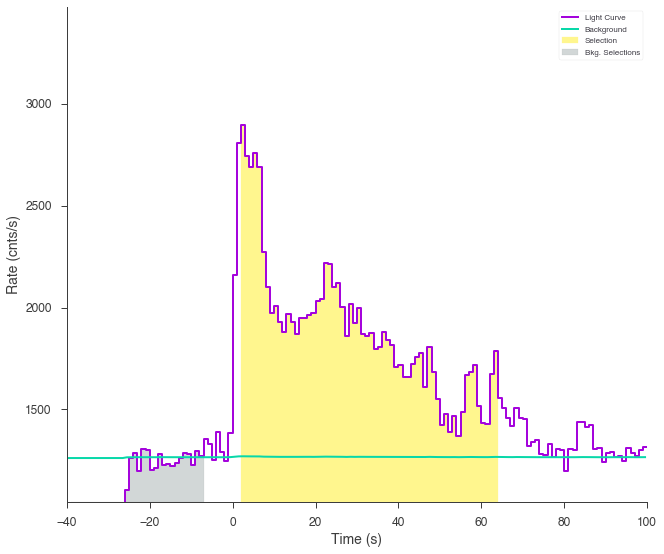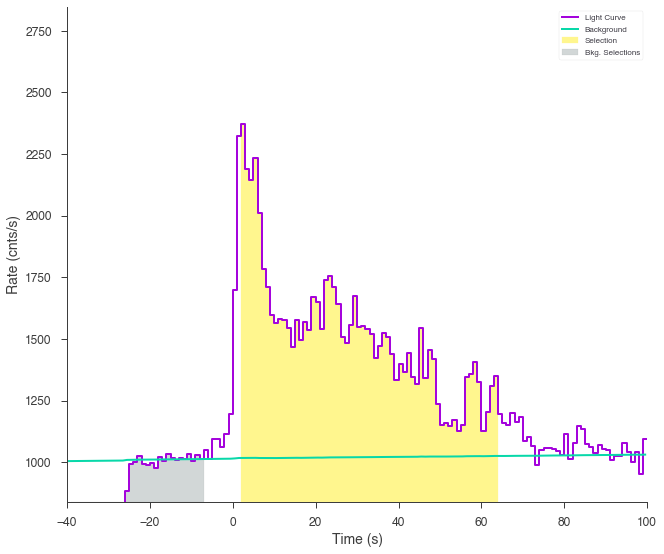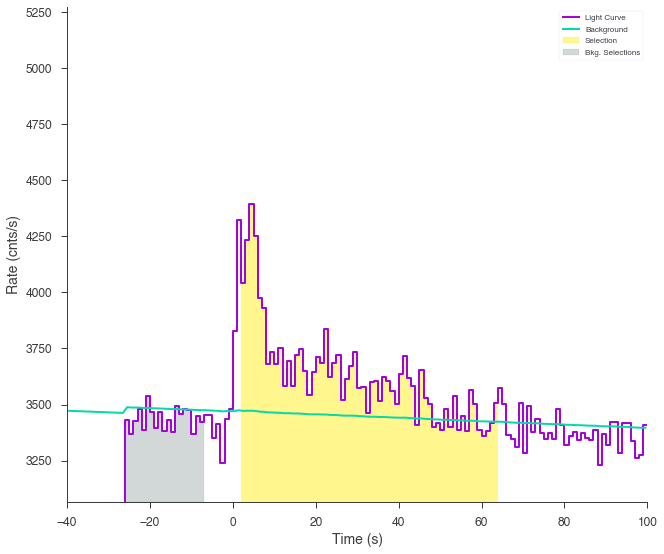## Setting up the fit¶

Let’s see if we can reproduce the results from the catalog.

### Set priors for the model¶

We will fit the spectrum using Bayesian analysis, so we must set priors on the model parameters.

:

model.GRB080916009.spectrum.main.shape.alpha.prior = Truncated_gaussian(lower_bound = -1.5, upper_bound = 1, mu=-1, sigma=0.5)
model.GRB080916009.spectrum.main.shape.beta.prior = Truncated_gaussian(lower_bound = -5, upper_bound = -1.6, mu=-2.25, sigma=0.5)
model.GRB080916009.spectrum.main.shape.break_energy.prior = Log_normal(mu=2, sigma=1)
model.GRB080916009.spectrum.main.shape.break_energy.bounds = (None, None)
model.GRB080916009.spectrum.main.shape.K.prior = Log_uniform_prior(lower_bound = 1E-3, upper_bound = 1E1)
model.GRB080916009.spectrum.main.shape.break_scale.prior = Log_uniform_prior(lower_bound = 1E-4, upper_bound = 10)


### Clone the model and setup the Bayesian analysis class¶

Next, we clone the model we built from the catalog so that we can look at the results later and fit the cloned model. We pass this model and the DataList of the plugins to a BayesianAnalysis class and set the sampler to MultiNest.

:

new_model = clone_model(model)

bayes = BayesianAnalysis(new_model, DataList(*fluence_plugins))
bayes.set_sampler("multinest")



### Examine at the catalog fitted model¶

We can quickly examine how well the catalog fit matches the data. There appears to be a discrepancy between the data and the model! Let’s refit to see if we can fix it.

:

display_spectrum_model_counts(bayes, min_rate=20, step=False );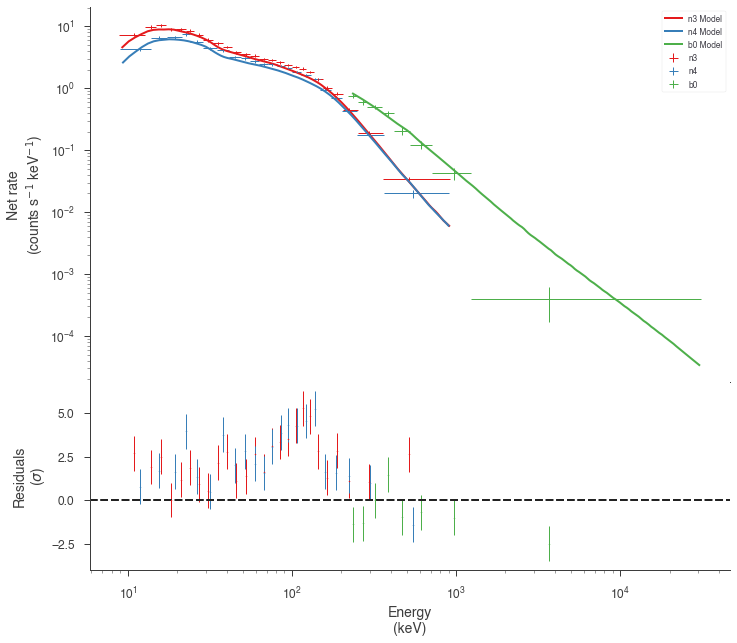### Run the sampler¶

We let MultiNest condition the model on the data

:

bayes.sampler.setup(n_live_points=400)
bayes.sample()

  analysing data from chains/fit-.txt
Maximum a posteriori probability (MAP) point:


result unit
parameter
GRB080916009...K (1.467 +/- 0.019) x 10^-2 1 / (cm2 keV s)
GRB080916009...alpha -1.070 -0.018 +0.019
GRB080916009...break_energy (2.38 -0.31 +0.32) x 10^2 keV
GRB080916009...break_scale (2.4 +/- 0.8) x 10^-1
GRB080916009...beta -2.22 +/- 0.12

Values of -log(posterior) at the minimum:


-log(posterior)
b0 -1072.839051
n3 -1023.565801
n4 -1014.475396
total -3110.880247

Values of statistical measures:


statistical measures
AIC 6231.930949
BIC 6251.163160
DIC 6218.937608
PDIC 4.569022
log(Z) -1356.935237

Now our model seems to match much better with the data!

:

bayes.restore_median_fit()
display_spectrum_model_counts(bayes, min_rate=20);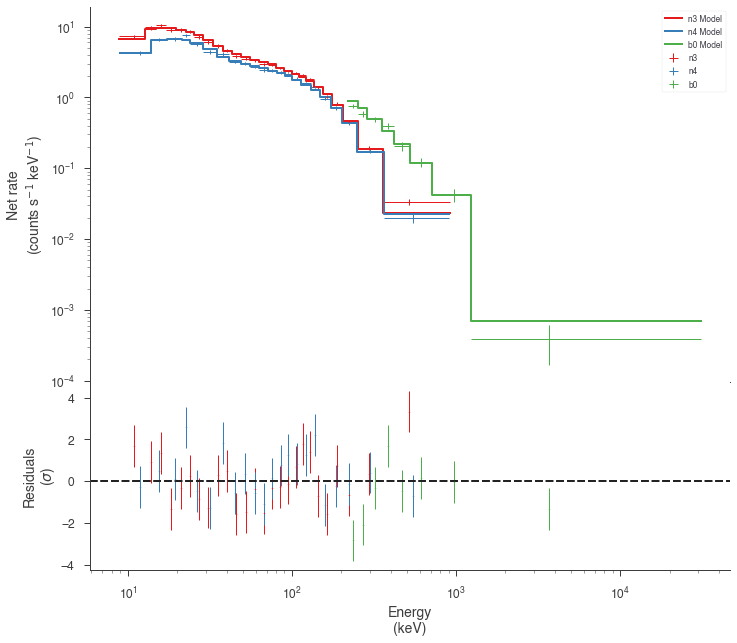But how different are we from the catalog model? Let’s plot our fit along with the catalog model. Luckily, 3ML can handle all the units for is

:

conversion = u.Unit('keV2/(cm2 s keV)').to('erg2/(cm2 s keV)')
energy_grid = np.logspace(1,4, 100)*u.keV
vFv = (energy_grid**2* model.get_point_source_fluxes(0, energy_grid)).to('erg2/(cm2 s keV)')


:

fig = plot_point_source_spectra(bayes.results, flux_unit='erg2/(cm2 s keV)');
ax = fig.get_axes()
ax.loglog(energy_grid, vFv, color='blue', label='catalog model')

:

[<matplotlib.lines.Line2D at 0x128e35d90>]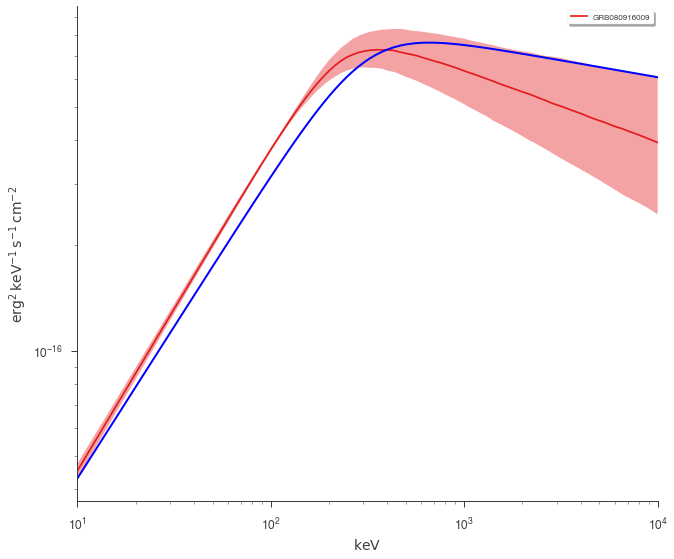## Time Resolved Analysis¶

Now that we have examined fluence fit, we can move to performing a time-resolved analysis.

### Selecting a temporal binning¶

We first get the brightest NaI detector and create time bins via the Bayesian blocks algorithm. We can use the fitted background to make sure that our intervals are chosen in an unbiased way.

:

n3 = time_series['n3']

:

n3.create_time_bins(0,60, method="bayesblocks", use_background=True, p0=0.2)

Created 15 bins via bayesblocks


Sometimes, glitches in the GBM data cause spikes in the data that the Bayesian blocks algorithm detects as fast changes in the count rate. We will have to remove those intervals manually.

...note In the future, 3ML will provide an automated method to remove these unwanted spikes.
:

n3.view_lightcurve(use_binner=True);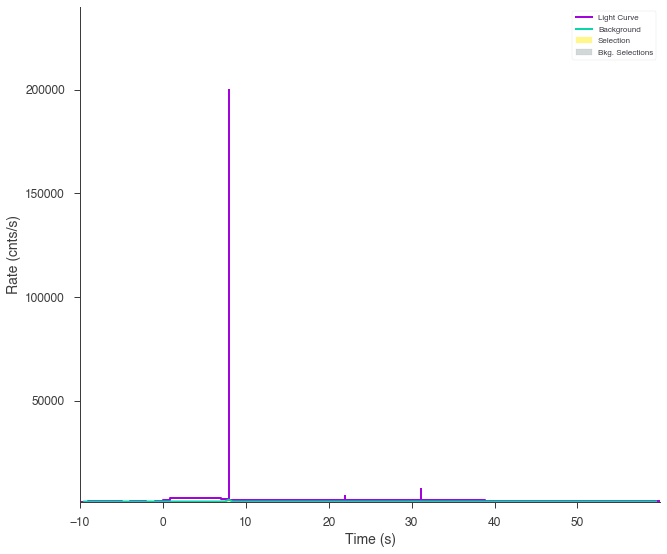:

bad_bins = []
for i, w in enumerate(n3.bins.widths):

if w < 5E-2:
bad_bins.append(i)

edges = [n3.bins.starts]

for i,b in enumerate(n3.bins):

if i not in bad_bins:
edges.append(b.stop)

starts=edges[:-1]
stops = edges[1:]

n3.create_time_bins(starts, stops, method='custom')

Created 12 bins via custom


Now our light curve looks much more acceptable.

:

n3.view_lightcurve(use_binner=True);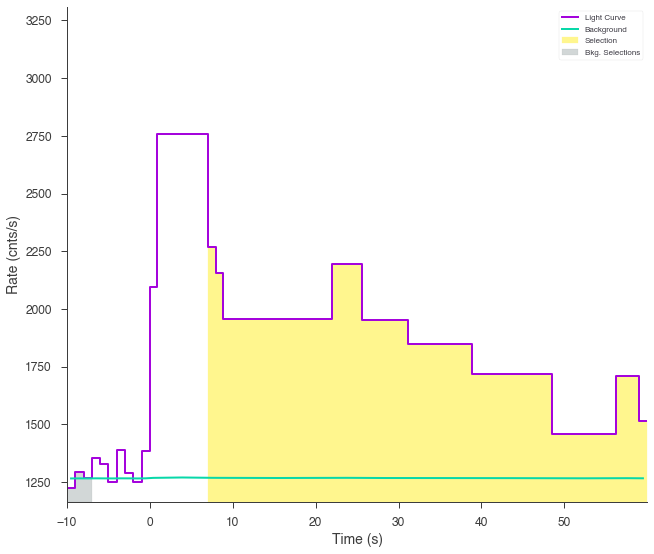The time series objects can read time bins from each other, so we will map these time bins onto the other detectors’ time series and create a list of time plugins for each detector and each time bin created above.

:

time_resolved_plugins = {}

for k,v in time_series.items():
v.read_bins(n3)
time_resolved_plugins[k] = v.to_spectrumlike(from_bins=True)

Created 12 bins via custom

Created 12 bins via custom

Created 12 bins via custom


### Setting up the model¶

For the time-resolved analysis, we will fit the classic Band function to the data. We will set some principled priors.

:

band = Band()
band.alpha.prior = Truncated_gaussian(lower_bound = -1.5, upper_bound = 1, mu=-1, sigma=0.5)
band.beta.prior = Truncated_gaussian(lower_bound = -5, upper_bound = -1.6, mu=-2, sigma=0.5)
band.xp.prior = Log_normal(mu=2, sigma=1)
band.xp.bounds = (0, None)
band.K.prior = Log_uniform_prior(lower_bound=1E-10, upper_bound=1E3)
ps = PointSource('grb', 0,0, spectral_shape=band)
band_model = Model(ps)



### Perform the fits¶

One way to perform Bayesian spectral fits to all the intervals is to loop through each one. There can are many ways to do this, so find an analysis pattern that works for you.

:

models = []
results = []
analysis = []
for interval in range(12):

# clone the model above so that we have a separate model
# for each fit

this_model = clone_model(band_model)

# for each detector set up the plugin
# for this time interval

this_data_list = []
for k, v in time_resolved_plugins.items():

pi = v[interval]

if k.startswith("b"):
pi.set_active_measurements("250-30000")
else:
pi.set_active_measurements("9-900")

pi.rebin_on_background(1.0)

this_data_list.append(pi)

# create a data list

dlist = DataList(*this_data_list)

# set up the sampler and fit

bayes = BayesianAnalysis(this_model, dlist)
bayes.set_sampler("multinest")
bayes.sampler.setup(n_live_points=500)
bayes.sample()

# at this stage we coudl also
# save the analysis result to
# disk but we will simply hold
# onto them in memory

analysis.append(bayes)

  analysing data from chains/fit-.txt
Maximum a posteriori probability (MAP) point:


result unit
parameter
grb.spectrum.main.Band.K (3.4 +/- 0.4) x 10^-2 1 / (cm2 keV s)
grb.spectrum.main.Band.alpha (-5.9 -0.9 +1.0) x 10^-1
grb.spectrum.main.Band.xp (3.5 +/- 0.5) x 10^2 keV
grb.spectrum.main.Band.beta -2.52 -0.20 +0.21

Values of -log(posterior) at the minimum:


-log(posterior)
b0_interval0 -284.092132
n3_interval0 -245.197184
n4_interval0 -262.694890
total -791.984205

Values of statistical measures:


statistical measures
AIC 1592.081725
BIC 1607.490543
DIC 1569.554646
PDIC 2.260288
log(Z) -345.419228
  analysing data from chains/fit-.txt
Maximum a posteriori probability (MAP) point:


result unit
parameter
grb.spectrum.main.Band.K (7.51 -0.18 +0.28) x 10^-2 1 / (cm2 keV s)
grb.spectrum.main.Band.alpha (-4.85 -0.22 +0.28) x 10^-1
grb.spectrum.main.Band.xp (2.376 -0.04 +0.031) x 10^2 keV
grb.spectrum.main.Band.beta -1.824 -0.010 +0.013

Values of -log(posterior) at the minimum:


-log(posterior)
b0_interval1 -699.207775
n3_interval1 -660.320455
n4_interval1 -650.387351
total -2009.915581

Values of statistical measures:


statistical measures
AIC 4027.944477
BIC 4043.353295
DIC 4006.031674
PDIC 1.310157
log(Z) -879.876570
  analysing data from chains/fit-.txt
Maximum a posteriori probability (MAP) point:


result unit
parameter
grb.spectrum.main.Band.K (2.92 -0.18 +0.19) x 10^-2 1 / (cm2 keV s)
grb.spectrum.main.Band.alpha -1.00 -0.05 +0.04
grb.spectrum.main.Band.xp (4.1 -0.8 +0.6) x 10^2 keV
grb.spectrum.main.Band.beta -1.84 -0.06 +0.05

Values of -log(posterior) at the minimum:


-log(posterior)
b0_interval2 -320.384149
n3_interval2 -282.657411
n4_interval2 -305.101494
total -908.143053

Values of statistical measures:


statistical measures
AIC 1824.399421
BIC 1839.808238
DIC 1800.887321
PDIC 1.397316
log(Z) -396.942679
  analysing data from chains/fit-.txt
Maximum a posteriori probability (MAP) point:


result unit
parameter
grb.spectrum.main.Band.K (3.10 -0.34 +0.33) x 10^-2 1 / (cm2 keV s)
grb.spectrum.main.Band.alpha (-8.7 +/- 0.8) x 10^-1
grb.spectrum.main.Band.xp (3.2 +/- 0.5) x 10^2 keV
grb.spectrum.main.Band.beta -2.43 +/- 0.30

Values of -log(posterior) at the minimum:


-log(posterior)
b0_interval3 -296.371175
n3_interval3 -237.332047
n4_interval3 -257.446049
total -791.149270

Values of statistical measures:


statistical measures
AIC 1590.411855
BIC 1605.820672
DIC 1568.567198
PDIC 2.532479
log(Z) -344.900925
  analysing data from chains/fit-.txt
Maximum a posteriori probability (MAP) point:


result unit
parameter
grb.spectrum.main.Band.K (2.10 +/- 0.09) x 10^-2 1 / (cm2 keV s)
grb.spectrum.main.Band.alpha (-9.62 -0.33 +0.32) x 10^-1
grb.spectrum.main.Band.xp (3.80 +/- 0.35) x 10^2 keV
grb.spectrum.main.Band.beta -2.03 +/- 0.08

Values of -log(posterior) at the minimum:


-log(posterior)
b0_interval4 -791.645837
n3_interval4 -751.711638
n4_interval4 -741.102354
total -2284.459829

Values of statistical measures:


statistical measures
AIC 4577.032972
BIC 4592.441790
DIC 4555.696500
PDIC 2.847320
log(Z) -995.361705
  analysing data from chains/fit-.txt
Maximum a posteriori probability (MAP) point:


result unit
parameter
grb.spectrum.main.Band.K (2.91 -0.20 +0.19) x 10^-2 1 / (cm2 keV s)
grb.spectrum.main.Band.alpha (-8.7 +/- 0.6) x 10^-1
grb.spectrum.main.Band.xp (3.9 +/- 0.5) x 10^2 keV
grb.spectrum.main.Band.beta -2.13 +/- 0.10

Values of -log(posterior) at the minimum:


-log(posterior)
b0_interval5 -541.990810
n3_interval5 -517.746571
n4_interval5 -521.703151
total -1581.440533

Values of statistical measures:


statistical measures
AIC 3170.994380
BIC 3186.403197
DIC 3149.682069
PDIC 3.257779
log(Z) -689.244839
  analysing data from chains/fit-.txt
Maximum a posteriori probability (MAP) point:


result unit
parameter
grb.spectrum.main.Band.K (2.03 -0.13 +0.12) x 10^-2 1 / (cm2 keV s)
grb.spectrum.main.Band.alpha (-9.9 +/- 0.5) x 10^-1
grb.spectrum.main.Band.xp (4.1 -0.5 +0.6) x 10^2 keV
grb.spectrum.main.Band.beta -2.56 -0.30 +0.33

Values of -log(posterior) at the minimum:


-log(posterior)
b0_interval6 -611.476875
n3_interval6 -578.625631
n4_interval6 -571.172859
total -1761.275365

Values of statistical measures:


statistical measures
AIC 3530.664045
BIC 3546.072862
DIC 3507.174576
PDIC 2.995084
log(Z) -766.890825
  analysing data from chains/fit-.txt
Maximum a posteriori probability (MAP) point:


result unit
parameter
grb.spectrum.main.Band.K (1.70 +/- 0.10) x 10^-2 1 / (cm2 keV s)
grb.spectrum.main.Band.alpha -1.03 +/- 0.04
grb.spectrum.main.Band.xp (4.1 +/- 0.5) x 10^2 keV
grb.spectrum.main.Band.beta -2.44 +/- 0.23

Values of -log(posterior) at the minimum:


-log(posterior)
b0_interval7 -670.570443
n3_interval7 -635.279771
n4_interval7 -644.799724
total -1950.649937

Values of statistical measures:


statistical measures
AIC 3909.413188
BIC 3924.822006
DIC 3886.444842
PDIC 2.968040
log(Z) -849.257030
  analysing data from chains/fit-.txt
Maximum a posteriori probability (MAP) point:


result unit
parameter
grb.spectrum.main.Band.K (1.58 +/- 0.13) x 10^-2 1 / (cm2 keV s)
grb.spectrum.main.Band.alpha (-8.2 +/- 0.6) x 10^-1
grb.spectrum.main.Band.xp (3.6 +/- 0.4) x 10^2 keV
grb.spectrum.main.Band.beta -2.57 -0.26 +0.27

Values of -log(posterior) at the minimum:


-log(posterior)
b0_interval8 -705.657496
n3_interval8 -693.385305
n4_interval8 -661.074788
total -2060.117589

Values of statistical measures:


statistical measures
AIC 4128.348492
BIC 4143.757309
DIC 4107.633785
PDIC 3.139963
log(Z) -896.859354
  analysing data from chains/fit-.txt
Maximum a posteriori probability (MAP) point:


result unit
parameter
grb.spectrum.main.Band.K (1.44 -0.7 +0.35) x 10^-2 1 / (cm2 keV s)
grb.spectrum.main.Band.alpha (-8.4 -2.3 +2.1) x 10^-1
grb.spectrum.main.Band.xp (1.2 +/- 0.4) x 10^2 keV
grb.spectrum.main.Band.beta -2.08 +/- 0.26

Values of -log(posterior) at the minimum:


-log(posterior)
b0_interval9 -654.621119
n3_interval9 -615.132418
n4_interval9 -614.107855
total -1883.861392

Values of statistical measures:


statistical measures
AIC 3775.836098
BIC 3791.244915
DIC 3720.126202
PDIC -41.553714
log(Z) -820.551581
  analysing data from chains/fit-.txt
Maximum a posteriori probability (MAP) point:


result unit
parameter
grb.spectrum.main.Band.K (2.3 +/- 0.5) x 10^-2 1 / (cm2 keV s)
grb.spectrum.main.Band.alpha (-6.8 +/- 1.3) x 10^-1
grb.spectrum.main.Band.xp (2.1 +/- 0.4) x 10^2 keV
grb.spectrum.main.Band.beta -2.03 +/- 0.14

Values of -log(posterior) at the minimum:


-log(posterior)
b0_interval10 -461.313070
n3_interval10 -433.623206
n4_interval10 -429.319522
total -1324.255798

Values of statistical measures:


statistical measures
AIC 2656.624911
BIC 2672.033728
DIC 2637.793545
PDIC 0.974562
log(Z) -577.201475
  analysing data from chains/fit-.txt
Maximum a posteriori probability (MAP) point:


result unit
parameter
grb.spectrum.main.Band.K (3.3 +/- 1.2) x 10^-2 1 / (cm2 keV s)
grb.spectrum.main.Band.alpha (-4.3 -2.2 +2.3) x 10^-1
grb.spectrum.main.Band.xp (1.30 -0.26 +0.27) x 10^2 keV
grb.spectrum.main.Band.beta -2.30 -0.32 +0.34

Values of -log(posterior) at the minimum:


-log(posterior)
b0_interval11 -291.851821
n3_interval11 -269.097236
n4_interval11 -252.570233
total -813.519291

Values of statistical measures:


statistical measures
AIC 1635.151896
BIC 1650.560713
DIC 1618.236530
PDIC 1.154841
log(Z) -354.525939

### Examine the fits¶

Now we can look at the fits in count space to make sure they are ok.

:

for a in analysis:
a.restore_median_fit()
display_spectrum_model_counts(a, min_rate=[20,20,-99], step=False)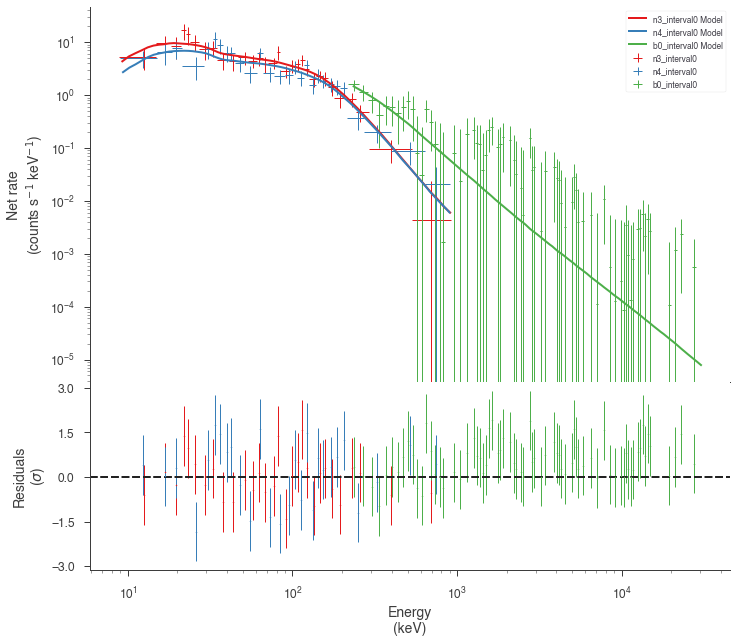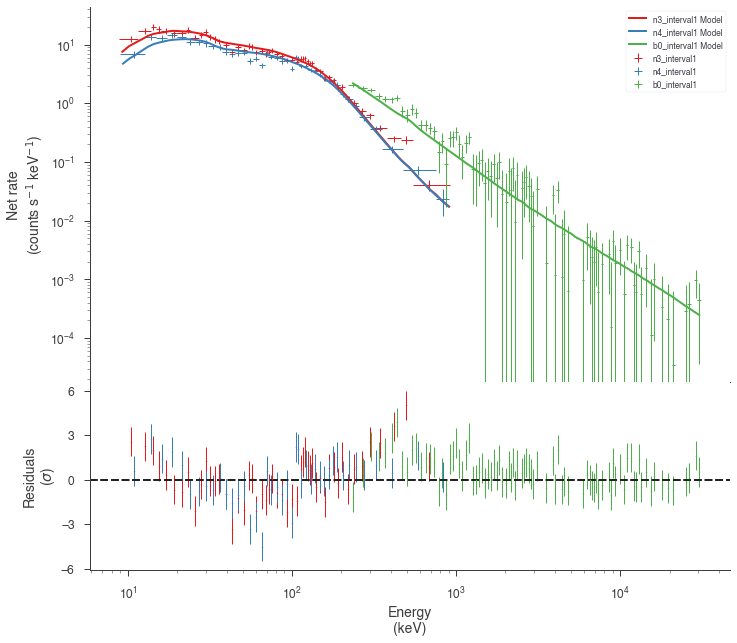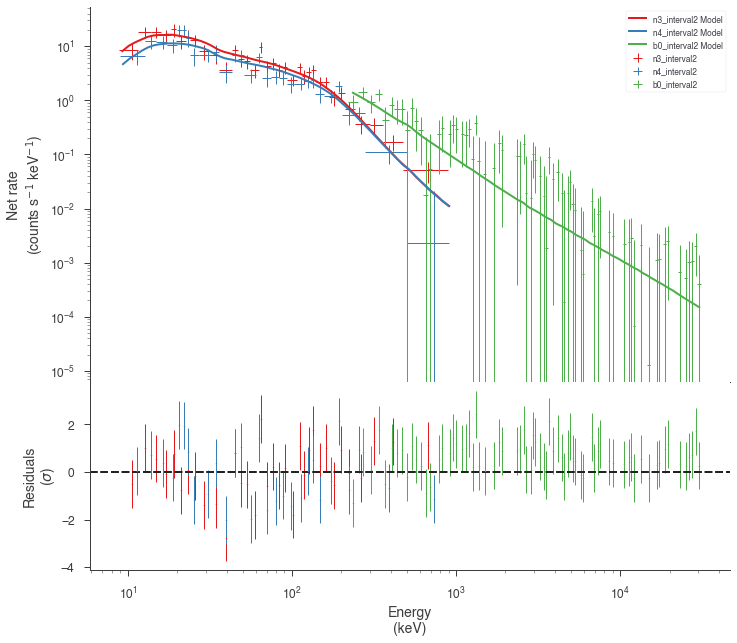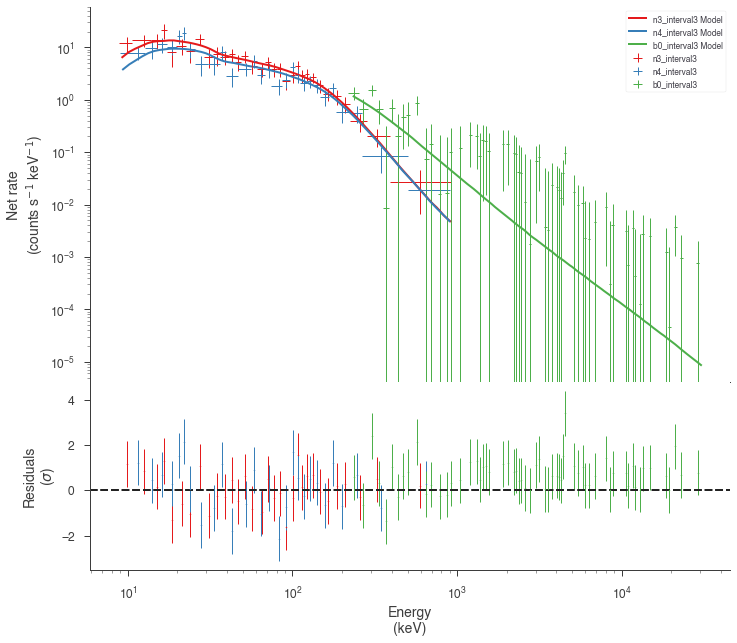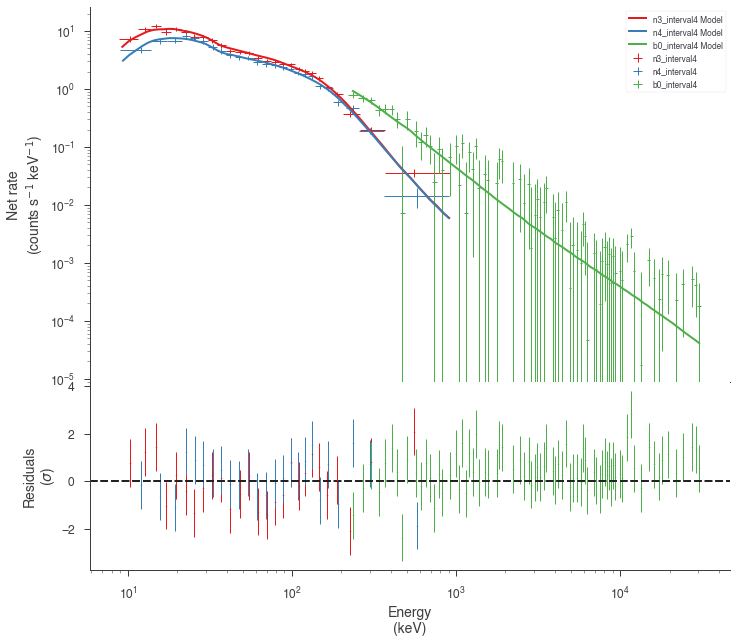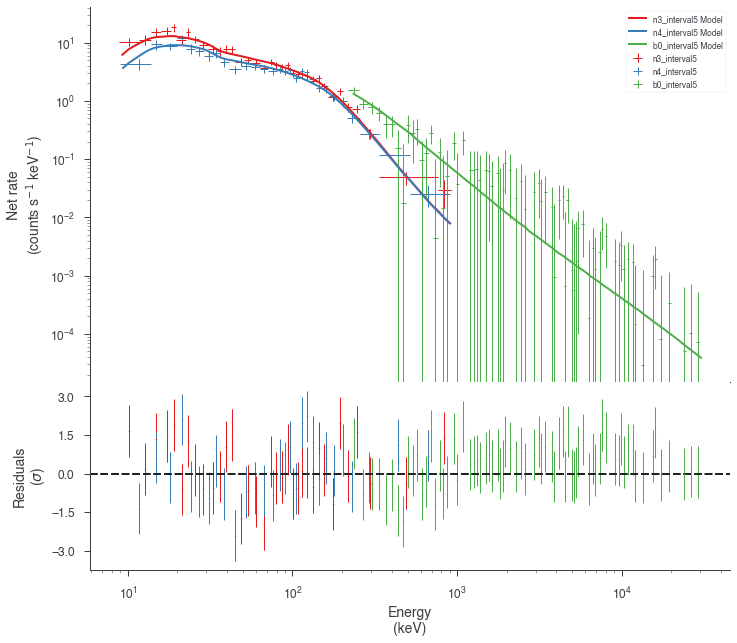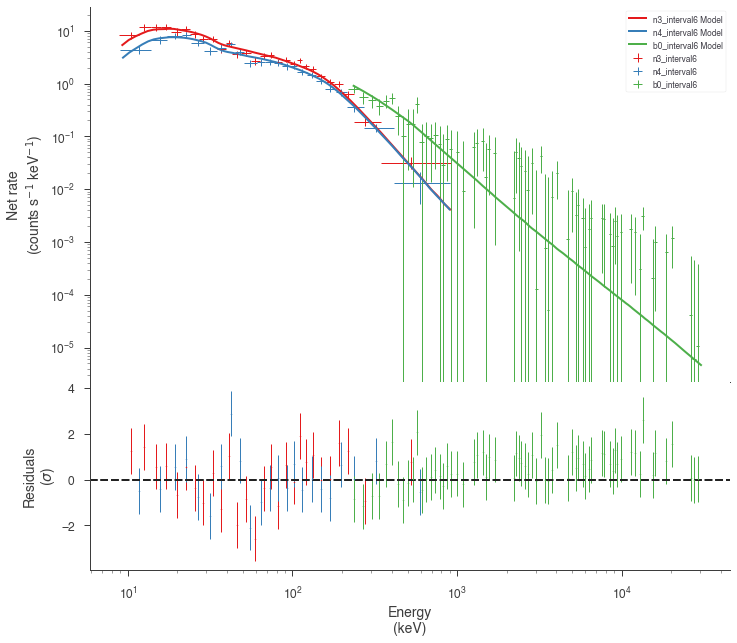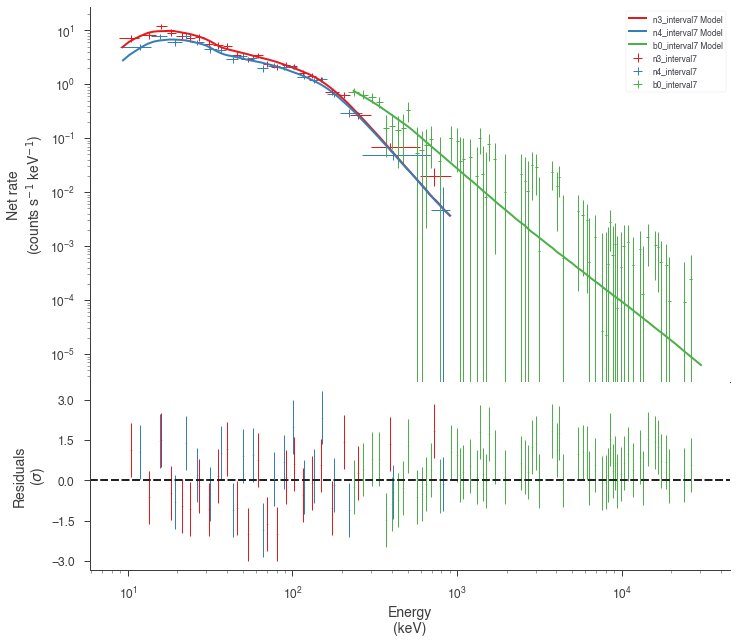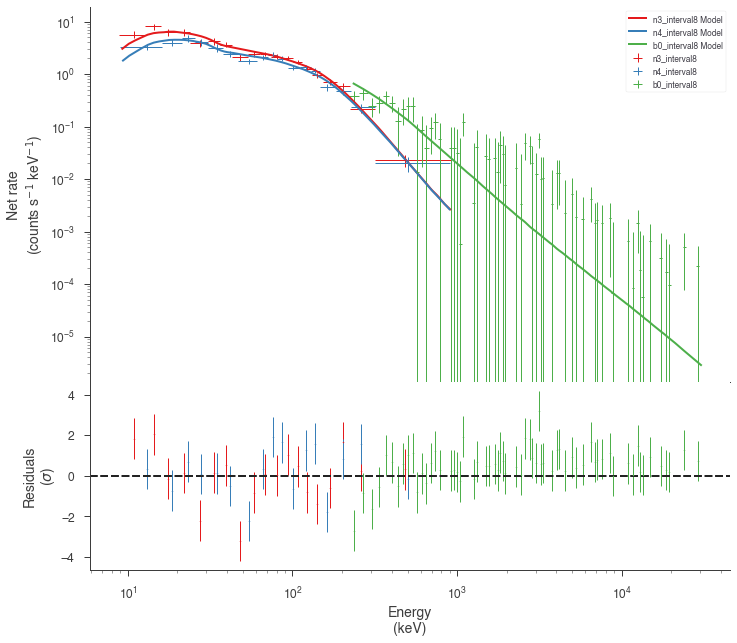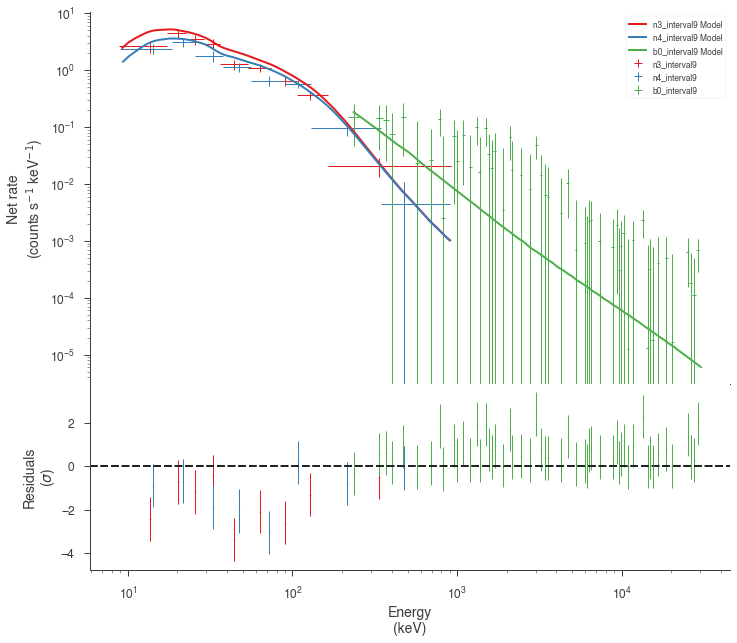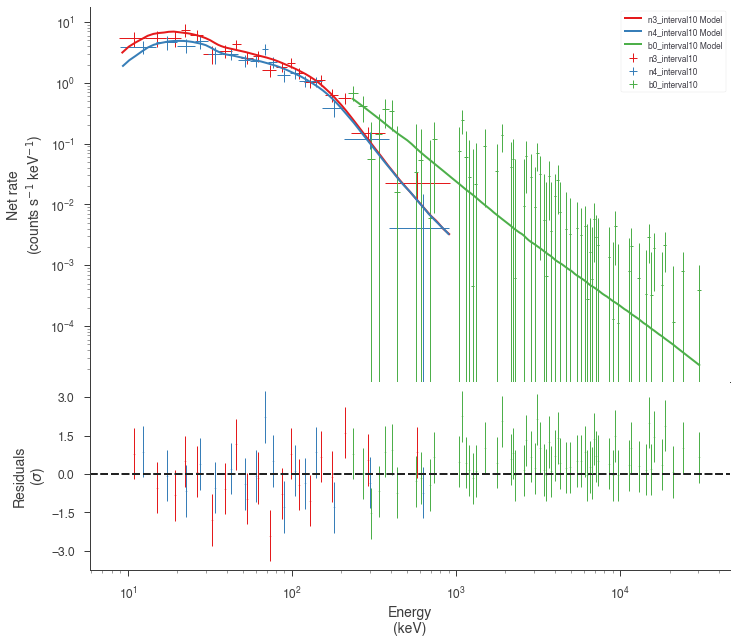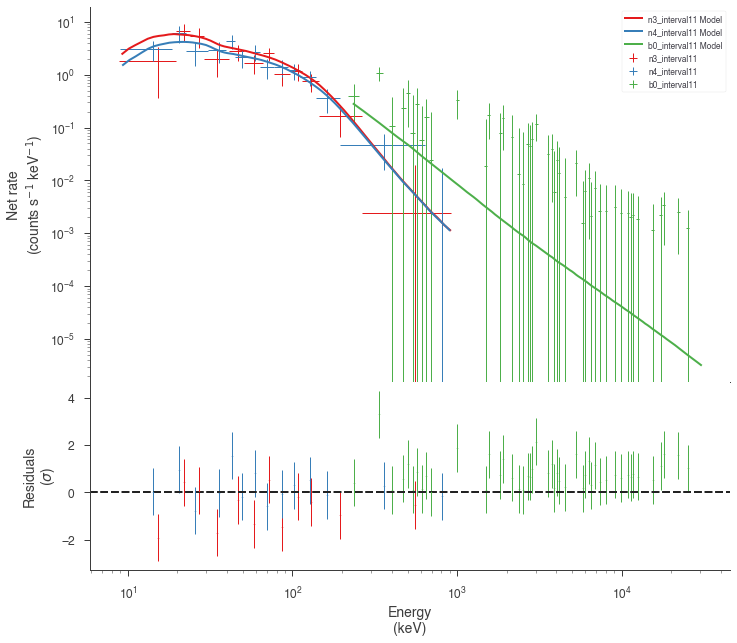Finally, we can plot the models together to see how the spectra evolve with time.

:

plot_spectra(*[a.results for a in analysis[::1]], flux_unit="erg2/(cm2 s keV)", fit_cmap='viridis', contour_cmap='viridis', contour_style_kwargs=dict(alpha=0.1));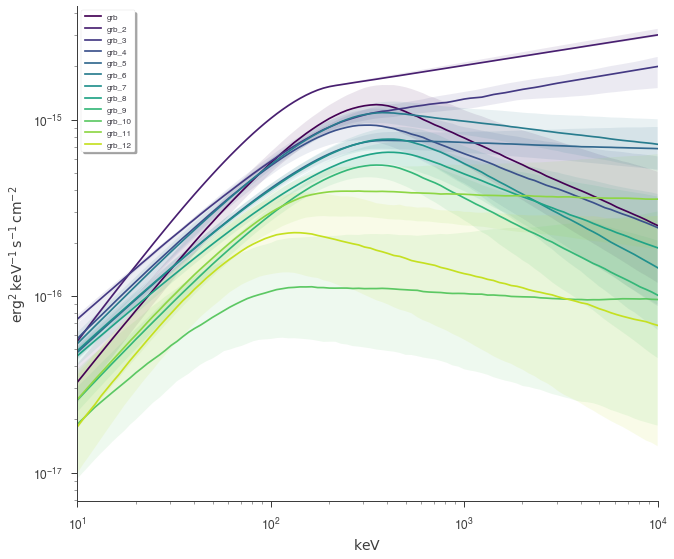This example can serve as a template for performing analysis on GBM data. However, as 3ML provides an abstract interface and modular building blocks, similar analysis pipelines can be built for any time series data.